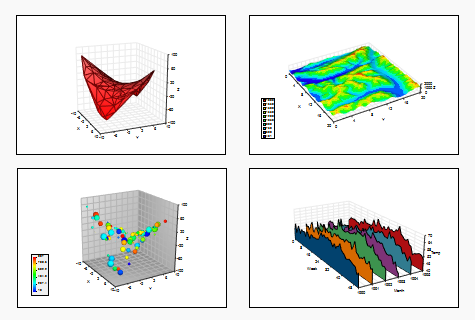T
TeraPlot

# 3D Graphing in TeraPlot

TeraPlot has a wide range of 3D plot types, most of which can be based on either mathematical expressions or tabulated data, and all of which can be combined within a single graph. For example, one or more planes, defined as surface plots, might be added to a scatter plot to segment the points according to some criterion. If also certain scatter points were required to be labelled, a 3D text plot could be added to label only the required points. This is just one example of the way that plot types can be combined to create composite visualizations. There are also a wide range of options for tailoring how the graph axes, walls and grid look. The list below summarises the plot types available in TeraPlot 3D graphing software. To see an example of a plot, press the magnifying glass icon adjacent to the plot title.

Home## Evaluate TeraPlot

TeraPlot is a Microsoft Windows desktop application (Windows XP, Vista, Windows 7, Windows 8 and 8.1). Download a fully functional 30-day evaluation version..

## Surface Plot - Regular Grid

A 3D surface is generated by associating a dependent variable value with each point on a grid with constant spacing in each independent variable direction. The grid can be cartesian, polar, cylindrical, or defined by parametric function coordinates (u, v). The plot can be defined analytically, based on entered/imported tabular data, or based on data read in from a file. The surface colour can be a single colour or based on a colourmap. By default, the colourmap colour displayed depends on surface height, but the option exists to use an arbitrary tabulated property to determine the colour associated with a surface point. A texture map (i.e an image) can optionally be overlaid on the surface. Further options include: transparency, contour display, general 3D transformation, wire frame display, features often only found in more expensive 3D graphing software.

## Surface Plot - Irregular Data

A 3D surface is generated by associating a dependent variable value with each of a set of points arbitrarily positioned in the independent variable plane. The plot can be defined analytically, or based on entered/imported tabular data. The surface colour can be a single colour or based on a colourmap. By default, the colourmap colour displayed depends on surface height, but the option exists to use an arbitrary tabulated property to determine the colour associated with a surface point. Further options include: transparency, general 3D transformation, wire frame display.

## Scatter Plot

Draws a symbol at each of a set of points in 3D space. The points can be defined in a cartesian, polar, or cylindrical coordinate system. TeraPlot 3D graphing software also allows up to two additional variables to be associated with each scatter point. These can then be visualised via the scatter point colour and/or size.

## Isosurface Plot

Draws surfaces of constant w for functions of the form w = f(x, y, z), i.e. the 3d equivalent of contour plots. The data from which the isosurfaces are generated can be defined as a formula (analytical plot), or loaded from a file. For an analytical plot, the data is assumed to lie on a regular rectangular 3D grid. For data loaded from a file, the data can either lie on a regular grid, or arbitrary individual cells can be specified.

## Bars - Regular Grid

Draws a bar of height z at each of the plot's (x,y) points. The points are constrained to lie on a rectangular grid with constant spacing in the x and y directions.

## Bars - Irregular Data

Draws a bar of height z at each of a given curve's (x,y) points. The points can be arbitrarily positioned in the (x, y) plane.

## Series Plot

Joins the (x, y, z) points in a plot by straight lines to form a series of (x, z) or (y, z) subcurves. The option exists to additionally draw a vertical sheet at each subcurve to produce a "waterfall" plot. The points are constrained to lie on a rectangular grid with constant spacing in the x and y directions.

## 3D Line

Joins a set of points in 3D space by straight lines. The points can be defined in a cartesian, polar (theta, phi or lat,lon), or cylindrical coordinate system.

## Wireframe Plot

A 3D see-through wireframe surface is generated by associating a dependent variable value with each point on a grid with constant spacing in each independent variable direction. The grid can be cartesian, polar, cylindrical, or defined by implicit function coordinates (u, v).

## Object Plot

Draws a set of 3D objects of predefined types. Current object types include sphere, cube and cylinder, The objects are described by TeraPlot Object Description Language (GODL). The description can be loaded from a file or or entered interactively.

## Mesh Plot

Draws a general 3D mesh defined as a set of vertices and elements read in from a file with data formatted in TeraPlot native ascii format. Variables can be associated with the mesh vertices or elements, and the mesh colourmapped based on these variables. The option also exists to load dxf files (FACE data only).

## Text Plot

Draws a piece of text at each of a set of points in 3D space. The text plot is usually used along with other plot types to provide annotation at specific points in a graph. The points can be defined in a cartesian, polar (theta, phi or lat,lon), or cylindrical coordinate system.

TeraPlot Graphing Software